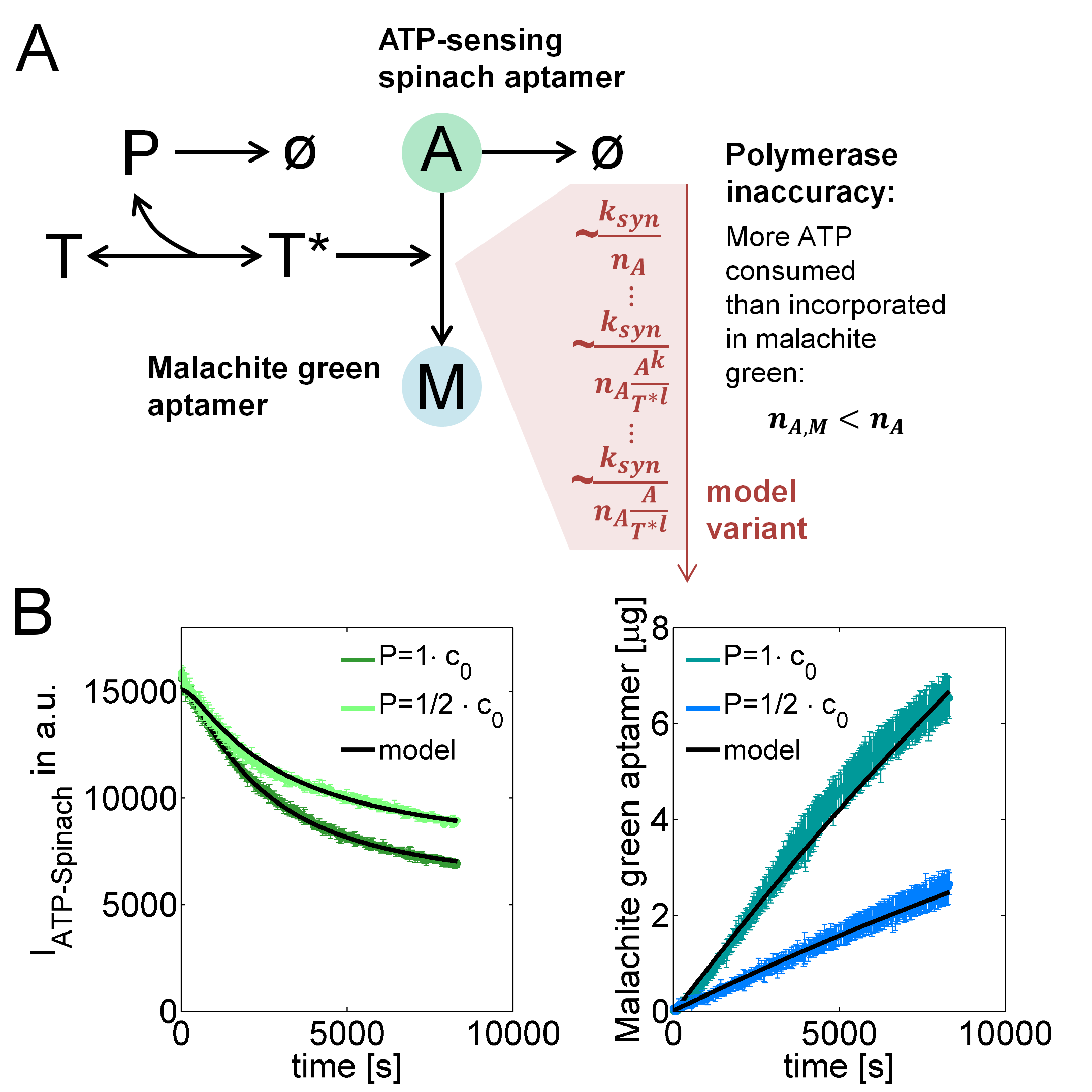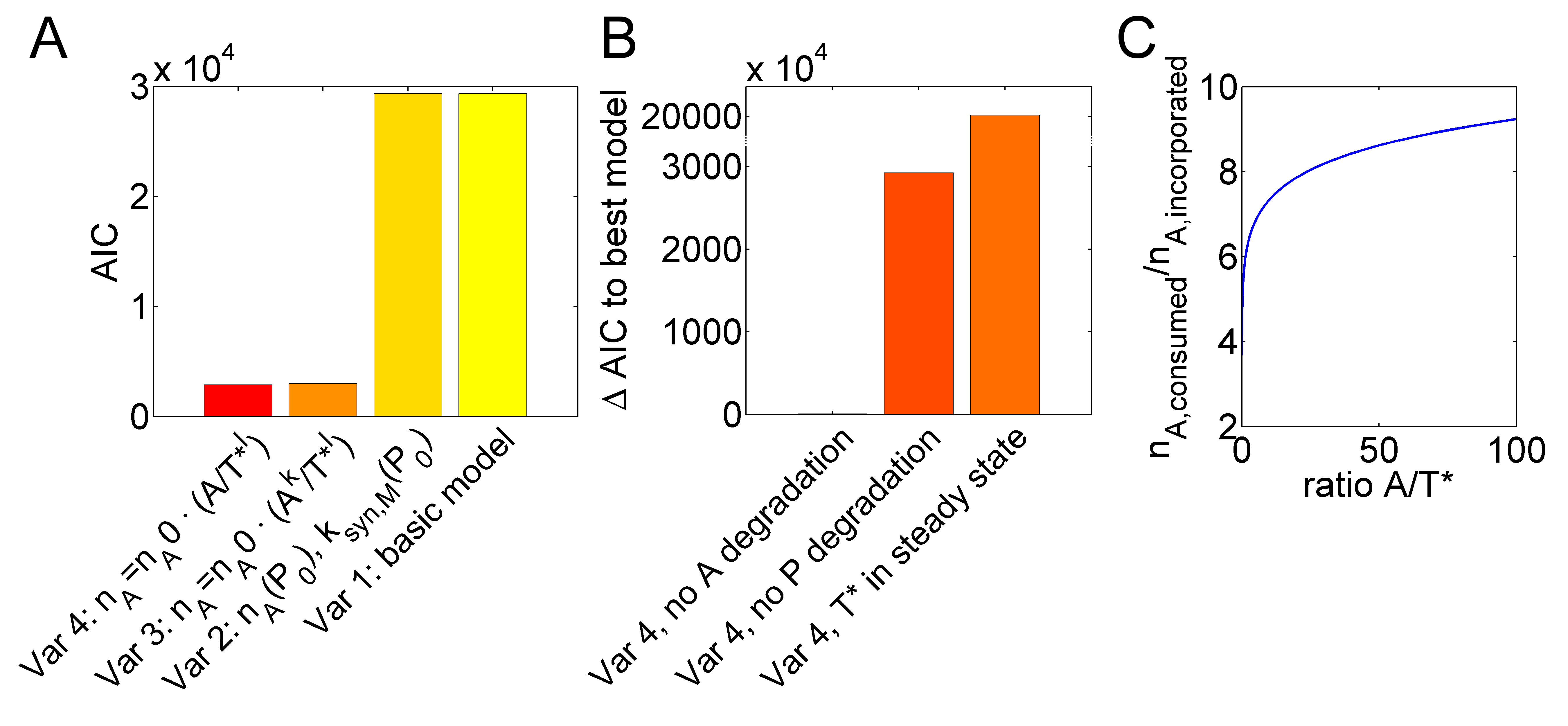# Team:Heidelberg/Modeling/rtsms### Studying determinants of polymerase efficiency based on an aptamer sensor

Our subproject on small molecule sensing facilitates quantitatively studying in vitro transcription (IVT) by ATP-spinach and malachite green RNA-aptamers. Here, we apply mathematical modeling to understand mechanistic details of this process and demonstrate that our approach can be used as a tool for basic research.

After adding an RNA polymerase to DNA templates, the polymerase binds to the template and starts consuming ATP by incorporating it into transcripts containing the malachite green aptamer. While the concentration of ATP could be monitored by fluorescence of the Spinach2-ATP-Aptamer, the transcript yield was monitored by malachite green fluorescence. This enabled us to follow IVT quantitatively and time-resolved. In particular, we could study the inaccuracy of polymerases reflected by an excess of consumed ATP molecules over the number of ATP molecules in synthesized malachite green aptamers.

To this end, we implemented a mathematical model that describes the formation of "active templates" $T^*$ from unbound DNA-templates $T$ and polymerases $P$, and the consumption of ATP $A$ for the synthesis of malachite green aptamers $M$ (Figure 1A). Because malachite green aptamers contain $n_{A,M}=10$ adenine nucleotides, the rate, at which malachite green is produced, is at least by this factor lower than the rate, at which ATP is consumed. The production of premature abortion products that result from the detachment of the polymerase from the template before completing the transcript, however, leads to an even larger number $n_A>n_{A,M}$. By calibrating the model with experimental data, we estimated this number to characterize this polymerase inaccuracy. For this purpose, we used datasets that were recorded with the T7 RNA polymerase. First, as depicted in Figure 1A, we tried to explain this inaccuracy by a constant number $n_A$ that was independent from DNA-template, ATP or polymerase concentrations. Then, we extended the model step-wise until the experimental data could be explained by the model. The step-wise extensions are listed in Table 1 while Table 2 contains the model equations for each variant.Figure 1. IVT model reactions and fits to experimental data. (A) Model reactions describing reversible assembly of templates $T$ and polymerase $P$ to active templates $T^*$ that incorporate ATP $A$ into malachite green RNA-aptamers $M$ but also into abortion products, leading to a higher number $n_A$ of consumed than ATP molecules $n_{A,M}$ incorporated in malachite green aptamers. (B) Model fits to data at two different polymerase concentrations.

Counter-intuitively, malachite green fluorescence showed a linear increase while the ATP-spinach fluorescence intensity was exponentially decreasing. Furthermore, it was surprising that doubling the amount of polymerase increased the production of malachite green by even more than two-fold. Because of these two unexpected findings, our basic model with constant values for $n_A$ could not explain the data. However, both phenomena could be explained by an optimal model variant (Figure 1B, 1A, Table 1), in which the polymerase inaccuracy increased with increasing ratios between ATP and active templates. Figure 2A visualizes the improvement in fit quality from the basic model to the optimal model variant (variant 4) in values of the Akaike information criterion (AIC) that accounts for the distance between the model and the experimental data and additionally penalizes for the number of model parameters to favor parsimonious model topologies.
Next, we tried if the optimal model, variant 4, could be simplified without losing fit quality. Leaving out degradation reactions for the polymerase $P$ strongly decreased fit quality (Figure 2B). Furthermore, assuming a fast binding of the polymerase to its template, which can be reflected in the model by a steady state of active template formation, resulted in a large AIC value increase. Leaving out ATP degradation, however, resulted only in a slight decrease in fit quality indicated by a small increase in the corresponding AIC value. We applied the rank-based Kruskal-Wallis test and found that, nevertheless, the small AIC value increase was significant ($p = 1.57\cdot10^{-4}$). This indicated that the optimal model could not be further reduced without losing fit quality. Essentially, in the optimal model variant, the rate of malachite green synthesis was dependent on a consumed number of ATP molecules $n_A=n_{A,0} A /T^{*l}$ for each malachite green aptamer molecule. In Figure 2C, the number $n_A$ is shown for different ratios between ATP and active template concentrations using the best fit parameters of the optimal model variant. The model thus predicts a high sensitivity of $n_A$ for changes of the $A /T^{*}$ ratio at values below $A /T^{*}\approx10$ and a low sensitivity of $n_A$ at higher ratios in the range above $A /T^{*}\approx30$ to $50$.Figure 2. IVT inaccuracy depends on the ATP to active template ratio. (A) A basic model with constant numbers of $n_A$ and synthesis parameters $k_{syn,M}$, was extended to variants with $n_A$ and $k_{syn,M}$ depending on the polymerase concentration (variant 2), $A$- and $T^*$-dependent $n_A$ with exponents $k$ and $l$ (variant 3) or only an exponent for $T^*$ (variant 4). Fitting improvement is indicated by decreasing Akaike information criterion (AIC) values. (B) Reducing the optimal variant 4 by assuming a steady state for $T^*$, no degradation of $P$ or no degradation of $A$ strongly worsened model fits. (C) Model variant 4 can explain increasing inefficiency (higher $n_A$) with decreasing $A/T^*$ ratios.

Taken together, our setup was suitable for studying the phenomenon of polymerase inaccuracy based on a mathematical model. We have learned that the inaccuracy of an RNA polymerase increases with an increasing ratio between ATP and active templates in a non-linear manner. Furthermore, we learned that the kinetics of polymerase binding to the DNA-template is relevant for the transcription dynamics. In the future, our approach might facilitate quantitative studies of the interaction between polymerases and promoters as well as the impact of DNA-modifications on the transcription dynamics.

Table 1. Stepwise changes from the basic model variant 1 to the optimal variant 4 and from variant 4 to variants 4a to 4c

 Model variant Subsequent modifications relative to the previous variant Changes in fitting quality 1 $k_{syn}$ and $n_A$ independent from polymerase concentrations 2 Individual $k_{syn}$ and $n_A$ values for different polymerase concentrations improvement 3 $n_A$ depends on function of $T^*$ and $A$ $n_A=n_{A,0} A^{k} /T^{*l}$ improvement, $k\approx0$ 4, best model Setting $k=0$ improvement 4a No degradation of $P$ in variant 4 decrease 4b No degradation of $A$ in variant 4 decrease 4c Binding of $P$ to $T$ in steady state in variant 4 decrease

Table 2. Model equations for the basic model and variants 1 to 4c

 Model species Variant Equation $P$ Variants 1 to 4, 4c $\frac{d[P]}{dt}=-k_{on}[T][P]+k_{off}[T^*]-k_{deg,P}[P]$ Variant 4a $[P](t)=[P](t_{0})\exp\left(-k_{deg,P}t\right)$ Variant 4b $\frac{d[P]}{dt}=-k_{on}[T][P]+k_{off}[T^*]$ $T$ Variants 1 to 4, 4b, 4c $\frac{d[T]}{dt}=-k_{on}[T][P]+k_{off}[T^*]$ Variant 4a $[T]=[T_{tot}]-[T^*]$ $T^*$ Variants 1 to 4, 4b, 4c $\frac{d[T^*]}{dt}=k_{on}[T][P]-k_{off}[T^*]$ Variant 4a $[T^*]=\frac{[T_{tot}][P]}{K_{d,P}}$ $A$ Variants 2 to 4, 4a, 4b $\frac{d[A]}{dt}=-k_{syn}[A][T^*]-k_{deg,A}[A]$ Variant 1 $\frac{d[A]}{dt}=-k_{syn}\frac{[A][T^*]}{K_{m,T}+[T^*]}-k_{deg,A}[A]$ Variant 4c $\frac{d[A]}{dt}=-k_{syn}[A][^*]$ $M$ Variant 2 $\frac{d[M]}{dt}=\frac{k_{syn}}{n_{A}}[A][T^*]$ Variants 1 $\frac{d[M]}{dt}=\frac{k_{syn}}{n_{A}}\frac{[A][T^*]}{K_{m,T}+[T^*]}$ Variant 3 $\frac{d[M]}{dt}=\frac{k_{syn}}{n_{A,0}\frac{[A]^{k}}{[T^*]^{l}}}[A][T^*]=\frac{k_{syn}}{n_{A,0}}[A]^{1-k}[T^*]^{1+j}$ Variants 4, 4a, 4b, 4c $\frac{d[M]}{dt}=\frac{k_{syn}}{n_{A,0}\frac{[A]}{[T*]^{l}}}[A][T^*]=\frac{k_{syn}}{n_{A,0}}[T^*]^{1+j}$